# SAT II Math II : Finding Angles

## Example Questions

2 Next →

### Example Question #361 : Sat Subject Test In Math Ii

Suppose a set of intersecting lines. If an angle is 120 degrees, what must be the sum of the adjacent angle and the vertical angle to the given angle?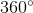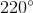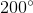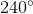Explanation:

In an intersecting pair of lines, recall that vertical angles will always equal.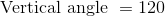The adjacent angle with the given angle will form a straight line, and both of the angles must sum to 180 degrees.

Subtract 120 from 180 to get the adjacent angle.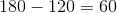Sum the two angles.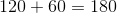The answer is:### Example Question #362 : Sat Subject Test In Math Ii

If a set of angles are supplementary, what must be the other angle if a given angle is?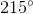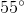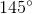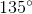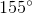Explanation:

Supplementary angles must add up to 180 degrees.

To find the missing angle, subtract the known angle from 180 degrees.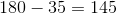The answer is:### Example Question #11 : Finding Angles

If two angles of a triangle are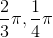radians, what must be the other angle in degrees?Explanation:

Every pi radians equal 180 degrees.

We can choose to convert the radians to degrees first.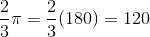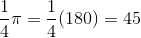The sum of these two angles are: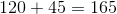Subtract this value fromto determine the third angle.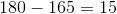The answer is:2 Next →